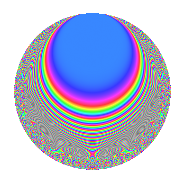# Properties

 Label 23.3.dLevel 23 Weight 3 Character orbit d Rep. character $$\chi_{23}(5,\cdot)$$ Character field $$\Q(\zeta_{22})$$ Dimension 30 Newforms 1 Sturm bound 6 Trace bound 0

# Related objects

## Defining parameters

 Level: $$N$$ = $$23$$ Weight: $$k$$ = $$3$$ Character orbit: $$[\chi]$$ = 23.d (of order $$22$$ and degree $$10$$) Character conductor: $$\operatorname{cond}(\chi)$$ = $$23$$ Character field: $$\Q(\zeta_{22})$$ Newforms: $$1$$ Sturm bound: $$6$$ Trace bound: $$0$$

## Dimensions

The following table gives the dimensions of various subspaces of $$M_{3}(23, [\chi])$$.

Total New Old
Modular forms 50 50 0
Cusp forms 30 30 0
Eisenstein series 20 20 0

## Trace form

 $$30q$$ $$\mathstrut -\mathstrut 11q^{2}$$ $$\mathstrut -\mathstrut 11q^{3}$$ $$\mathstrut -\mathstrut 23q^{4}$$ $$\mathstrut -\mathstrut 11q^{5}$$ $$\mathstrut +\mathstrut 22q^{6}$$ $$\mathstrut -\mathstrut 11q^{7}$$ $$\mathstrut +\mathstrut 10q^{8}$$ $$\mathstrut -\mathstrut 38q^{9}$$ $$\mathstrut +\mathstrut O(q^{10})$$ $$30q$$ $$\mathstrut -\mathstrut 11q^{2}$$ $$\mathstrut -\mathstrut 11q^{3}$$ $$\mathstrut -\mathstrut 23q^{4}$$ $$\mathstrut -\mathstrut 11q^{5}$$ $$\mathstrut +\mathstrut 22q^{6}$$ $$\mathstrut -\mathstrut 11q^{7}$$ $$\mathstrut +\mathstrut 10q^{8}$$ $$\mathstrut -\mathstrut 38q^{9}$$ $$\mathstrut -\mathstrut 11q^{10}$$ $$\mathstrut -\mathstrut 11q^{11}$$ $$\mathstrut -\mathstrut 14q^{12}$$ $$\mathstrut -\mathstrut 11q^{13}$$ $$\mathstrut -\mathstrut 11q^{14}$$ $$\mathstrut +\mathstrut 66q^{15}$$ $$\mathstrut +\mathstrut 73q^{16}$$ $$\mathstrut +\mathstrut 44q^{17}$$ $$\mathstrut +\mathstrut 126q^{18}$$ $$\mathstrut +\mathstrut 22q^{19}$$ $$\mathstrut +\mathstrut 77q^{20}$$ $$\mathstrut +\mathstrut 22q^{21}$$ $$\mathstrut +\mathstrut 36q^{23}$$ $$\mathstrut -\mathstrut 22q^{24}$$ $$\mathstrut -\mathstrut 152q^{25}$$ $$\mathstrut -\mathstrut 186q^{26}$$ $$\mathstrut -\mathstrut 62q^{27}$$ $$\mathstrut -\mathstrut 275q^{28}$$ $$\mathstrut -\mathstrut 88q^{29}$$ $$\mathstrut -\mathstrut 363q^{30}$$ $$\mathstrut -\mathstrut 110q^{31}$$ $$\mathstrut -\mathstrut 147q^{32}$$ $$\mathstrut -\mathstrut 132q^{33}$$ $$\mathstrut +\mathstrut 231q^{34}$$ $$\mathstrut +\mathstrut 209q^{35}$$ $$\mathstrut +\mathstrut 229q^{36}$$ $$\mathstrut +\mathstrut 341q^{37}$$ $$\mathstrut +\mathstrut 374q^{38}$$ $$\mathstrut +\mathstrut 295q^{39}$$ $$\mathstrut +\mathstrut 429q^{40}$$ $$\mathstrut +\mathstrut 77q^{41}$$ $$\mathstrut +\mathstrut 319q^{42}$$ $$\mathstrut +\mathstrut 77q^{43}$$ $$\mathstrut +\mathstrut 110q^{44}$$ $$\mathstrut -\mathstrut 99q^{46}$$ $$\mathstrut -\mathstrut 110q^{47}$$ $$\mathstrut -\mathstrut 550q^{48}$$ $$\mathstrut -\mathstrut 422q^{49}$$ $$\mathstrut -\mathstrut 396q^{50}$$ $$\mathstrut -\mathstrut 275q^{51}$$ $$\mathstrut -\mathstrut 472q^{52}$$ $$\mathstrut -\mathstrut 187q^{53}$$ $$\mathstrut -\mathstrut 198q^{54}$$ $$\mathstrut -\mathstrut 165q^{55}$$ $$\mathstrut +\mathstrut 176q^{56}$$ $$\mathstrut -\mathstrut 176q^{57}$$ $$\mathstrut -\mathstrut 13q^{58}$$ $$\mathstrut -\mathstrut q^{59}$$ $$\mathstrut +\mathstrut 539q^{60}$$ $$\mathstrut +\mathstrut 297q^{61}$$ $$\mathstrut +\mathstrut 82q^{62}$$ $$\mathstrut +\mathstrut 264q^{63}$$ $$\mathstrut +\mathstrut 386q^{64}$$ $$\mathstrut +\mathstrut 220q^{65}$$ $$\mathstrut +\mathstrut 264q^{66}$$ $$\mathstrut +\mathstrut 11q^{67}$$ $$\mathstrut -\mathstrut 66q^{69}$$ $$\mathstrut -\mathstrut 198q^{70}$$ $$\mathstrut -\mathstrut 176q^{71}$$ $$\mathstrut -\mathstrut 605q^{72}$$ $$\mathstrut -\mathstrut 121q^{73}$$ $$\mathstrut -\mathstrut 352q^{74}$$ $$\mathstrut +\mathstrut 154q^{75}$$ $$\mathstrut +\mathstrut 110q^{76}$$ $$\mathstrut +\mathstrut 110q^{77}$$ $$\mathstrut +\mathstrut 360q^{78}$$ $$\mathstrut +\mathstrut 33q^{79}$$ $$\mathstrut -\mathstrut 242q^{80}$$ $$\mathstrut +\mathstrut 494q^{81}$$ $$\mathstrut +\mathstrut 96q^{82}$$ $$\mathstrut -\mathstrut 154q^{83}$$ $$\mathstrut +\mathstrut 11q^{84}$$ $$\mathstrut +\mathstrut 275q^{85}$$ $$\mathstrut +\mathstrut 143q^{86}$$ $$\mathstrut +\mathstrut 271q^{87}$$ $$\mathstrut +\mathstrut 429q^{88}$$ $$\mathstrut +\mathstrut 121q^{89}$$ $$\mathstrut +\mathstrut 242q^{90}$$ $$\mathstrut +\mathstrut 166q^{92}$$ $$\mathstrut +\mathstrut 260q^{93}$$ $$\mathstrut -\mathstrut 295q^{94}$$ $$\mathstrut -\mathstrut 154q^{95}$$ $$\mathstrut -\mathstrut 419q^{96}$$ $$\mathstrut +\mathstrut 154q^{97}$$ $$\mathstrut +\mathstrut 77q^{98}$$ $$\mathstrut -\mathstrut 242q^{99}$$ $$\mathstrut +\mathstrut O(q^{100})$$

## Decomposition of $$S_{3}^{\mathrm{new}}(23, [\chi])$$ into irreducible Hecke orbits

Label Dim. $$A$$ Field CM Traces $q$-expansion
$$a_2$$ $$a_3$$ $$a_5$$ $$a_7$$
23.3.d.a $$30$$ $$0.627$$ None $$-11$$ $$-11$$ $$-11$$ $$-11$$